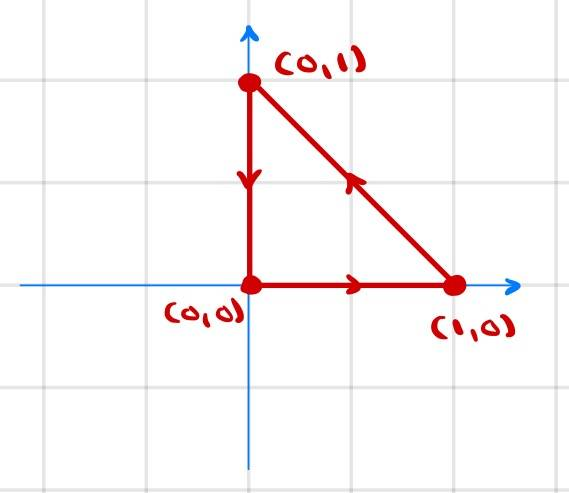# Apostol, Ch. 10, Problem about line integrals -- Is the book answer correct?

zenterix
Homework Statement:
Calculate the line integral with respect to arc length.

##\int_C (x+y)ds##, where ##C## is the triangle with vertices ##(0,0), (1,0)## and ##(0,1)##, traversed in a counterclockwise direction.
Relevant Equations:
There are three separate paths on the triangle with parametric equations

$$\vec{r_1}(t)=\langle t,0 \rangle$$
$$\vec{r_2}(t)=\langle 1-t,t \rangle$$
$$\vec{r_3}(t)=\langle 0,1-t \rangle$$

The corresponding velocity vectors are
$$\vec{v_1}(t)=\langle 1,0 \rangle$$
$$\vec{v_2}(t)=\langle -1,1 \rangle$$
$$\vec{v_3}(t)=\langle 0,-1 \rangle$$

and speeds

$$v_1(t)=1$$
$$v_2(t)=\sqrt{2}$$
$$v_3(t)=1$$

The line integral then becomes

$$\int_C (x+y)ds = \int_0^1 t dt+\int_0^1 \sqrt{2} dt+\int_0^1 (1-t)dt$$

$$=1/2+\sqrt{2}+1-1/2$$

$$=\sqrt{2}+1$$
The answer at the end of the book says ##-\sqrt{2}##.

Is this correct or is my solution correct?

Here is a depiction of the path where we are integratingHomework Helper
Gold Member
We can see the book solution is wrong without doing any integrating. The integrand ##(x+y)## is positive everywhere along the line of integration, and ##ds## is always positive by definition. So the integral has to be positive. So it can't be ##-\sqrt 2##.

•jim mcnamara, zenterix and FactChecker
Homework Helper
Gold Member
2022 Award
Maybe we are missing some context here.
The diagram shows a specified path around the loop. The given answer works if we allow ds to be signed, e.g. going from (0,1) to (0,0) giving the opposite sign to the reverse direction. That would be how a line integral works in a vector field or in the complex plane. And complex analysis is what I associate with the name Apostol.

Homework Helper
Gold Member
Maybe we are missing some context here.
The diagram shows a specified path around the loop. The given answer works if we allow ds to be signed, e.g. going from (0,1) to (0,0) giving the opposite sign to the reverse direction. That would be how a line integral works in a vector field or in the complex plane. And complex analysis is what I associate with the name Apostol.
My interpretation of ds would be a change of length in a parameterized path. So the counter-clockwise path has a positive ds going down the Y-axis.

Homework Helper
2022 Award
This is exercise 7 in section 10.9 of Apostol, Calculus Vol II (2nd ed). For context, on pages 329-330, the following definition is given:

Let $\alpha$ be a path with $\alpha'$ continuous on $[a,b]$. The graph of $\alpha$ is a rectifiable curve. In Volume I we proved that the corresponding arc length integral is given by $$s(t) = \int_a^t \|\alpha'(u)\|\,du$$ The derivative of arc length is given by $$s'(t) = \|\alpha'(t)\|.$$ Let $\phi$ be a scalar field, defined and bounded on $C$, the graph of $\alpha$. The line integral of $\phi$ with respect to arc length along $C$ is given by $$\int_C \phi\,ds = \int_a^b \phi(\alpha(t))s'(t)\,dt,$$ whenever the integral on the right exists.

The OP's calculations would therefore appear to be correct, and the answer given in the book is wrong. Searching for errata turned up this exchange from 2015:

I am studying calculus using apostol, I found that some answers at the back are wrong. I tried to search for an errata copy but can't find any. Does anyone know how to get one or has a list of errors?

I would contact the publisher

I should note that I did check Wiley's website, but no errata are given there.

•FactChecker
•